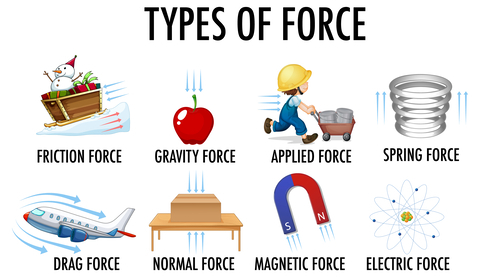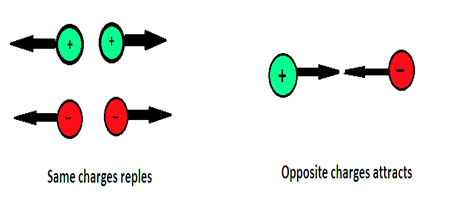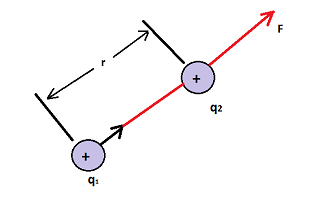# Electrical Force

## Introduction

Electromagnetism is one of the most important branches of physics. Among the forces we see in our daily life, all the forces except gravity are electromagnetic. All the forces we encounter in life except gravity (including the tension of a wire, the vertical force of a surface, and the force of friction) are electromagnetic forces that appear between atoms.Any neutral substance has equal number of electrons and protons. If the outermost electrons leave the atom, it becomes free electron and causes an electric current. An atom that has lost an outer electron has a higher positive charge. When one object rubs against another, particles migrate from one object to another.

## Electrical Force

The electric force between charges is of two types.

One is attractive and the other is repulsive. The same charges repel each other; Opposite charges attract each other. The force between electric charges is called electric force. This force belongs to the type of non-contact force. Because this motion works without the charges touching each other. The area around a charge that can feel its charge is called an electric field. The direction of the force acting on a small positive charge is calledthe direction of the electric field and represented by the lines of force. Lines of charge are straight or curved lines drawn in the direction in which a uniform electric charge tends to move in an electric field. They are imaginary lines.

Fig 2. Properties of Charges

## How would you define an Electric Force?

The electric force is directly related to the product of the charges and inversely related to the square of the distance between them. The electrostatic force between two point charges is governed by Newton’s third law. A force on one charge acts as a reaction and a force on another charge acts as the opposite reaction.

## What does the size of Electric Force depend on?

The electric force depends on the distance between any two charge points and the product of the charges on the point particles. But in Newton’s third law, the gravitational force is equivalent to the product of the point masses. So, there is some important difference between Newton’s third law and Coulomb’s law.

## What is Coulomb’s Law?The electric force is directly proportional to the product of the charge of the particles and inversely proportional to the square of the distance between them.

Fig 3. Coulomb force between two points

$$\mathrm{F\:\alpha\:\frac{{q_1}{q_2}}{r^2}}$$

In 1786, Coulomb presented the equation for the force between two stationary point electric charges in free space. Consider two stationary point particles separated by a distance r in a vacuum with charges $\mathrm{q_{1}}$ and $\mathrm{q_{2}}$ respectively. According to this law, the force acting on a point charge $\mathrm{q_{1}}$ on a point charge $\mathrm{q_{2}}$ is written as

$$\mathrm{\overrightarrow{F_{21}}=k\frac{q_{1}q_{2}}{r^{2}}\widehat{r_{12}}}$$

Where $\mathrm{\widehat{r_{12}}}$ is the univariate vector drawn from $\mathrm{q_{1}}$ to $\mathrm{q_{2}}$ and k is the adaptive constant.

### Important properties

• Coulomb’s law has the same structure as Newton’s law of gravitation. In both cases, the force is inversely related to the the distance.

• The gravitational force between two masses is always attractive. The Coulomb’s force is either an attractive or repulsive force depending on the nature of the particle.

• The value of the gravitational constant is $\mathrm{G=6.626\times 10^{-11}Nm^{2}kg^{-2}}$. But the value of the Coulomb’s constant $\mathrm{k=9\times 10^{9}Nm^{2}C^{-2}}$.‘k’ in Coulomb's law is much greater than the value of G. So the value of the electrostatic force is much greater than the gravitational force for an object with less mass.

• The gravitational force remains the same whether the masses are stationary or in motion. But, when the particles move, another force (Lawrence force) starts acting along with the Coulomb force.

• Coulomb’s law applies only to point-charge particles. But point particles are just a concept. Practically impossible. If the size of the particles is very small compared to the distance between them, we can use this law.

• The gravitational force between two masses is independent of the medium in which they are placed. For example, the value of the gravitational force acting between two 1 kg masses does not change regardless of whether they are placed in air or water. But the electrostatic force acting between two electrons depends on the nature of the medium in which they are placed.

## What is the formula for electric force?

Coulomb law gives the formula for the electric force which is given by

$$\mathrm{F\propto \frac{q_{1}q_{2}}{r^{2}}}$$

$$\mathrm{F=k \frac{q_{1}q_{2}}{r^{2}}}$$

$$\mathrm{F=\frac{1}{4\pi \epsilon _{0}} \frac{q_{1}q_{2}}{r^{2}}}$$

F - Electric force

$\mathrm{q_{1}q_{2}}$ - Charges of the particles

k - constant($\mathrm{\frac{1}{4\pi \epsilon _{0}}}$)

r - the distance between the charges

## Electrical Force Examples

• About two thousand years ago, the ancient Greeks discovered that a substance called amber ( a translucent, fossilized tree sap) rubbed with animal fur or wool attracted leaves and dust. So amber has the characteristics of charge. That is, it produces electrical force when it is rubbed by other things.

• When dry hair is combed and brought near a small piece of paper, it becomes charged. A ballon rubbed on our hand sticks to the wall without any friction. In all the above operations, when one substance rubs the surface of another substance, they become charged and attract each other by the electric force.

• A glass rod rubbed with silk was also found to attract pieces of paper. Thus, even a glass rod rubbed with a suitable material is charged. It attracts the paper by the electric force.

• When a charged glass rod is brought near a rubbed rod, they attract each other. At the same time, if a charged glass rod is brought close to another charged glass rod, they will be seen to repel each other. The following conclusions can be drawn from these observations.

• The charge received by the rubber rod is different from the charge received by the glass rod.

• A charged rubber rod repels another charged rubber rod. From this, it can be said that like charges repel each other.

• A charged rubber rod attracts a charged glass rod. From this, it is known that the charge on the glass rod and the charge on the sphere are not of the same type and that different charges attract each other.

## Conclusion

The atom was known to be electrically neutral through the pioneering studies of scientists such as J.J.Thomson and A.Rutherford who carried out their research in the late 19th and early 20th centuries. All the forces we encounter in life except gravity (including the tension of a wire, the vertical force of a surface, and the force of friction) are electromagnetic forces that appear between atoms. The electric force between charges is of two types. One is attractive and the other is repulsive. Same charges repel each other; Opposite charges attract each other. The force between electric charges is called electric force. This force belongs to the type of non-contact force. In 1786, Coulomb presented the equation for the force between two stationary point electric charges in free space.

## FAQs

Q1. Define emf.

Ans. Batteries and other sources of electrical energy act as a resistor, pushing charges so that they flow through a wire or conductor. This pushing action of power sources is done by their electromotive force (emf). In an electrical circuit, it is equivalent to the work done by a unit charge once it travels around the circuit.

Q2. What are electric field lines?

Ans. Electric field lines are a series of lines drawn to show the electric field at a point in space. Electric field lines start at positive charges and end at opposite charges or infinity.

Q3.Explain the principle of microwave oven

Ans. A microwave oven works on the principle of torque acting on an electric dipole. We know that the water molecules in the food we eat are stable electric dipoles. The microwave produced by this product is oscillating electromagnetic waves. Hence they exert a twisting force on the water molecules.

Q4. Why copper wires are used in household electrical circuits?

Ans. Copper conductors have very low resistance. For this reason, copper wires are used in household electrical circuits. Materials surround these wires with high electrical resistance.

Q5. Give an example of a magnetic field

Ans. Place a magnet on the table and place some iron paper clips near it. Move the magnet slowly towards the paper clips at a point where the paper clips stick to the magnet. From the above function, we observe that magnets have an invisible field around them.